# Style Transfer¶

In this notebook we will implement the style transfer technique from "Image Style Transfer Using Convolutional Neural Networks" (Gatys et al., CVPR 2015).

The general idea is to take two images, and produce a new image that reflects the content of one but the artistic "style" of the other. We will do this by first formulating a loss function that matches the content and style of each respective image in the feature space of a deep network, and then performing gradient descent on the pixels of the image itself.

The deep network we use as a feature extractor is SqueezeNet, a small model that has been trained on ImageNet. You could use any network, but we chose SqueezeNet here for its small size and efficiency.

Here's an example of the images you'll be able to produce by the end of this notebook:## Setup¶

In :
import torch
import torch.nn as nn
import torchvision
import torchvision.transforms as T
import PIL

import numpy as np

from collections import namedtuple
import matplotlib.pyplot as plt

from cs231n.image_utils import SQUEEZENET_MEAN, SQUEEZENET_STD
%matplotlib inline


We provide you with some helper functions to deal with images, since for this part of the assignment we're dealing with real JPEGs, not CIFAR-10 data.

In :
def preprocess(img, size=512):
transform = T.Compose([
T.Scale(size),
T.ToTensor(),
T.Normalize(mean=SQUEEZENET_MEAN.tolist(),
std=SQUEEZENET_STD.tolist()),
T.Lambda(lambda x: x[None]),
])
return transform(img)

def deprocess(img):
transform = T.Compose([
T.Lambda(lambda x: x),
T.Normalize(mean=[0, 0, 0], std=[1.0 / s for s in SQUEEZENET_STD.tolist()]),
T.Normalize(mean=[-m for m in SQUEEZENET_MEAN.tolist()], std=[1, 1, 1]),
T.Lambda(rescale),
T.ToPILImage(),
])
return transform(img)

def rescale(x):
low, high = x.min(), x.max()
x_rescaled = (x - low) / (high - low)
return x_rescaled

def rel_error(x,y):
return np.max(np.abs(x - y) / (np.maximum(1e-8, np.abs(x) + np.abs(y))))

def features_from_img(imgpath, imgsize):
img = preprocess(PIL.Image.open(imgpath), size=imgsize)
img_var = Variable(img.type(dtype))
return extract_features(img_var, cnn), img_var

# Older versions of scipy.misc.imresize yield different results
# from newer versions, so we check to make sure scipy is up to date.
def check_scipy():
import scipy
vnum = int(scipy.__version__.split('.'))
assert vnum >= 16, "You must install SciPy >= 0.16.0 to complete this notebook."

check_scipy()



As in the last assignment, we need to set the dtype to select either the CPU or the GPU

In :
dtype = torch.FloatTensor
# Uncomment out the following line if you're on a machine with a GPU set up for PyTorch!
# dtype = torch.cuda.FloatTensor

In :
# Load the pre-trained SqueezeNet model.
cnn = torchvision.models.squeezenet1_1(pretrained=True).features
cnn.type(dtype)

# We don't want to train the model any further, so we don't want PyTorch to waste computation
# computing gradients on parameters we're never going to update.
for param in cnn.parameters():

# We provide this helper code which takes an image, a model (cnn), and returns a list of
# feature maps, one per layer.
def extract_features(x, cnn):
"""
Use the CNN to extract features from the input image x.

Inputs:
- x: A PyTorch Variable of shape (N, C, H, W) holding a minibatch of images that
will be fed to the CNN.
- cnn: A PyTorch model that we will use to extract features.

Returns:
- features: A list of feature for the input images x extracted using the cnn model.
features[i] is a PyTorch Variable of shape (N, C_i, H_i, W_i); recall that features
from different layers of the network may have different numbers of channels (C_i) and
spatial dimensions (H_i, W_i).
"""
features = []
prev_feat = x
for i, module in enumerate(cnn._modules.values()):
next_feat = module(prev_feat)
features.append(next_feat)
prev_feat = next_feat
return features


## Computing Loss¶

We're going to compute the three components of our loss function now. The loss function is a weighted sum of three terms: content loss + style loss + total variation loss. You'll fill in the functions that compute these weighted terms below.

## Content loss¶

We can generate an image that reflects the content of one image and the style of another by incorporating both in our loss function. We want to penalize deviations from the content of the content image and deviations from the style of the style image. We can then use this hybrid loss function to perform gradient descent not on the parameters of the model, but instead on the pixel values of our original image.

Let's first write the content loss function. Content loss measures how much the feature map of the generated image differs from the feature map of the source image. We only care about the content representation of one layer of the network (say, layer $\ell$), that has feature maps $A^\ell \in \mathbb{R}^{1 \times C_\ell \times H_\ell \times W_\ell}$. $C_\ell$ is the number of filters/channels in layer $\ell$, $H_\ell$ and $W_\ell$ are the height and width. We will work with reshaped versions of these feature maps that combine all spatial positions into one dimension. Let $F^\ell \in \mathbb{R}^{N_\ell \times M_\ell}$ be the feature map for the current image and $P^\ell \in \mathbb{R}^{N_\ell \times M_\ell}$ be the feature map for the content source image where $M_\ell=H_\ell\times W_\ell$ is the number of elements in each feature map. Each row of $F^\ell$ or $P^\ell$ represents the vectorized activations of a particular filter, convolved over all positions of the image. Finally, let $w_c$ be the weight of the content loss term in the loss function.

Then the content loss is given by:

$L_c = w_c \times \sum_{i,j} (F_{ij}^{\ell} - P_{ij}^{\ell})^2$

In :
def content_loss(content_weight, content_current, content_original):
"""
Compute the content loss for style transfer.

Inputs:
- content_weight: Scalar giving the weighting for the content loss.
- content_current: features of the current image; this is a PyTorch Tensor of shape
(1, C_l, H_l, W_l).
- content_target: features of the content image, Tensor with shape (1, C_l, H_l, W_l).

Returns:
- scalar content loss
"""
content_loss = content_weight*torch.sum((torch.pow(content_current-content_original,2)))
return content_loss


Test your content loss. You should see errors less than 0.001.

In :
def content_loss_test(correct):
content_image = 'styles/tubingen.jpg'
image_size =  192
content_layer = 3
content_weight = 6e-2

c_feats, content_img_var = features_from_img(content_image, image_size)

student_output = content_loss(content_weight, c_feats[content_layer], feats[content_layer]).data.numpy()
error = rel_error(correct, student_output)
print('Maximum error is {:.6f}'.format(error))


Maximum error is 0.000176


## Style loss¶

Now we can tackle the style loss. For a given layer $\ell$, the style loss is defined as follows:

First, compute the Gram matrix G which represents the correlations between the responses of each filter, where F is as above. The Gram matrix is an approximation to the covariance matrix -- we want the activation statistics of our generated image to match the activation statistics of our style image, and matching the (approximate) covariance is one way to do that. There are a variety of ways you could do this, but the Gram matrix is nice because it's easy to compute and in practice shows good results.

Given a feature map $F^\ell$ of shape $(1, C_\ell, M_\ell)$, the Gram matrix has shape $(1, C_\ell, C_\ell)$ and its elements are given by:

$$G_{ij}^\ell = \sum_k F^{\ell}_{ik} F^{\ell}_{jk}$$

Assuming $G^\ell$ is the Gram matrix from the feature map of the current image, $A^\ell$ is the Gram Matrix from the feature map of the source style image, and $w_\ell$ a scalar weight term, then the style loss for the layer $\ell$ is simply the weighted Euclidean distance between the two Gram matrices:

$$L_s^\ell = w_\ell \sum_{i, j} \left(G^\ell_{ij} - A^\ell_{ij}\right)^2$$

In practice we usually compute the style loss at a set of layers $\mathcal{L}$ rather than just a single layer $\ell$; then the total style loss is the sum of style losses at each layer:

$$L_s = \sum_{\ell \in \mathcal{L}} L_s^\ell$$

Begin by implementing the Gram matrix computation below:

In :
def gram_matrix(features, normalize=True):
"""
Compute the Gram matrix from features.

Inputs:
- features: PyTorch Variable of shape (N, C, H, W) giving features for
a batch of N images.
- normalize: optional, whether to normalize the Gram matrix
If True, divide the Gram matrix by the number of neurons (H * W * C)

Returns:
- gram: PyTorch Variable of shape (N, C, C) giving the
(optionally normalized) Gram matrices for the N input images.
"""
N, C, H, W = features.size()
# Use torch.bmm for batch multiplication of matrices
feat_reshaped = features.view(N, C, -1)
gram = torch.bmm(feat_reshaped, feat_reshaped.transpose(1,2))
if normalize:
return gram/(H*W*C)
else:
return gram


Test your Gram matrix code. You should see errors less than 0.001.

In :
def gram_matrix_test(correct):
style_image = 'styles/starry_night.jpg'
style_size = 192
feats, _ = features_from_img(style_image, style_size)
student_output = gram_matrix(feats.clone()).data.numpy()
error = rel_error(correct, student_output)
print('Maximum error is {:.5f}'.format(error))


Maximum error is 0.00041


Next, implement the style loss:

In :
# Now put it together in the style_loss function...
def style_loss(feats, style_layers, style_targets, style_weights):
"""
Computes the style loss at a set of layers.

Inputs:
- feats: list of the features at every layer of the current image, as produced by
the extract_features function.
- style_layers: List of layer indices into feats giving the layers to include in the
style loss.
- style_targets: List of the same length as style_layers, where style_targets[i] is
a PyTorch Variable giving the Gram matrix the source style image computed at
layer style_layers[i].
- style_weights: List of the same length as style_layers, where style_weights[i]
is a scalar giving the weight for the style loss at layer style_layers[i].

Returns:
- style_loss: A PyTorch Variable holding a scalar giving the style loss.
"""
# Hint: you can do this with one for loop over the style layers, and should
# not be very much code (~5 lines). You will need to use your gram_matrix function.
style_loss = Variable(torch.FloatTensor())
for i in range(len(style_layers)):
gram = gram_matrix(feats[style_layers[i]])
style_loss += style_weights[i]*torch.sum(torch.pow(gram-style_targets[i], 2))
return style_loss


Test your style loss implementation. The error should be less than 0.001.

In :
def style_loss_test(correct):
content_image = 'styles/tubingen.jpg'
style_image = 'styles/starry_night.jpg'
image_size =  192
style_size = 192
style_layers = [1, 4, 6, 7]
style_weights = [300000, 1000, 15, 3]

c_feats, _ = features_from_img(content_image, image_size)
feats, _ = features_from_img(style_image, style_size)
style_targets = []
for idx in style_layers:
style_targets.append(gram_matrix(feats[idx].clone()))

student_output = style_loss(c_feats, style_layers, style_targets, style_weights).data.numpy()
error = rel_error(correct, student_output)
print('Error is {:.3f}'.format(error))


Error is 0.000


## Total-variation regularization¶

It turns out that it's helpful to also encourage smoothness in the image. We can do this by adding another term to our loss that penalizes wiggles or "total variation" in the pixel values.

You can compute the "total variation" as the sum of the squares of differences in the pixel values for all pairs of pixels that are next to each other (horizontally or vertically). Here we sum the total-variation regualarization for each of the 3 input channels (RGB), and weight the total summed loss by the total variation weight, $w_t$:

$L_{tv} = w_t \times \sum_{c=1}^3\sum_{i=1}^{H-1} \sum_{j=1}^{W-1} \left( (x_{i,j+1, c} - x_{i,j,c})^2 + (x_{i+1, j,c} - x_{i,j,c})^2 \right)$

In the next cell, fill in the definition for the TV loss term. To receive full credit, your implementation should not have any loops.

In :
def tv_loss(img, tv_weight):
"""
Compute total variation loss.

Inputs:
- img: PyTorch Variable of shape (1, 3, H, W) holding an input image.
- tv_weight: Scalar giving the weight w_t to use for the TV loss.

Returns:
- loss: PyTorch Variable holding a scalar giving the total variation loss
for img weighted by tv_weight.
"""
# Your implementation should be vectorized and not require any loops!
w_variance = torch.sum(torch.pow(img[:,:,:,:-1]-img[:,:,:,1:],2))
h_variance = torch.sum(torch.pow(img[:,:,:-1,:]-img[:,:,1:,:],2))
loss = tv_weight*(h_variance+w_variance)
return loss


Test your TV loss implementation. Error should be less than 0.001.

In :
def tv_loss_test(correct):
content_image = 'styles/tubingen.jpg'
image_size =  192
tv_weight = 2e-2

content_img = preprocess(PIL.Image.open(content_image), size=image_size)
content_img_var = Variable(content_img.type(dtype))

student_output = tv_loss(content_img_var, tv_weight).data.numpy()
error = rel_error(correct, student_output)
print('Error is {:.6f}'.format(error))


Error is 0.000406


Now we're ready to string it all together (you shouldn't have to modify this function):

In :
def style_transfer(content_image, style_image, image_size, style_size, content_layer, content_weight,
style_layers, style_weights, tv_weight, init_random = False):
"""
Run style transfer!

Inputs:
- content_image: filename of content image
- style_image: filename of style image
- image_size: size of smallest image dimension (used for content loss and generated image)
- style_size: size of smallest style image dimension
- content_layer: layer to use for content loss
- content_weight: weighting on content loss
- style_layers: list of layers to use for style loss
- style_weights: list of weights to use for each layer in style_layers
- tv_weight: weight of total variation regularization term
- init_random: initialize the starting image to uniform random noise
"""

# Extract features for the content image
content_img = preprocess(PIL.Image.open(content_image), size=image_size)
content_img_var = Variable(content_img.type(dtype))
feats = extract_features(content_img_var, cnn)
content_target = feats[content_layer].clone()

# Extract features for the style image
style_img = preprocess(PIL.Image.open(style_image), size=style_size)
style_img_var = Variable(style_img.type(dtype))
feats = extract_features(style_img_var, cnn)
style_targets = []
for idx in style_layers:
style_targets.append(gram_matrix(feats[idx].clone()))

# Initialize output image to content image or nois
if init_random:
img = torch.Tensor(content_img.size()).uniform_(0, 1)
else:
img = content_img.clone().type(dtype)

# We do want the gradient computed on our image!

# Set up optimization hyperparameters
initial_lr = 3.0
decayed_lr = 0.1
decay_lr_at = 180

# Note that we are optimizing the pixel values of the image by passing
# in the img_var Torch variable, whose requires_grad flag is set to True

f, axarr = plt.subplots(1,2)
axarr.axis('off')
axarr.axis('off')
axarr.set_title('Content Source Img.')
axarr.set_title('Style Source Img.')
axarr.imshow(deprocess(content_img.cpu()))
axarr.imshow(deprocess(style_img.cpu()))
plt.show()
plt.figure()

for t in range(200):
if t < 190:
img.clamp_(-1.5, 1.5)

feats = extract_features(img_var, cnn)

# Compute loss
c_loss = content_loss(content_weight, feats[content_layer], content_target)
s_loss = style_loss(feats, style_layers, style_targets, style_weights)
t_loss = tv_loss(img_var, tv_weight)
loss = c_loss + s_loss + t_loss

loss.backward()

# Perform gradient descents on our image values
if t == decay_lr_at:
optimizer.step()

if t % 100 == 0:
print('Iteration {}'.format(t))
plt.axis('off')
plt.imshow(deprocess(img.cpu()))
plt.show()
print('Iteration {}'.format(t))
plt.axis('off')
plt.imshow(deprocess(img.cpu()))
plt.show()


## Generate some pretty pictures!¶

Try out style_transfer on the three different parameter sets below. Make sure to run all three cells. Feel free to add your own, but make sure to include the results of style transfer on the third parameter set (starry night) in your submitted notebook.

• The content_image is the filename of content image.
• The style_image is the filename of style image.
• The image_size is the size of smallest image dimension of the content image (used for content loss and generated image).
• The style_size is the size of smallest style image dimension.
• The content_layer specifies which layer to use for content loss.
• The content_weight gives weighting on content loss in the overall loss function. Increasing the value of this parameter will make the final image look more realistic (closer to the original content).
• style_layers specifies a list of which layers to use for style loss.
• style_weights specifies a list of weights to use for each layer in style_layers (each of which will contribute a term to the overall style loss). We generally use higher weights for the earlier style layers because they describe more local/smaller scale features, which are more important to texture than features over larger receptive fields. In general, increasing these weights will make the resulting image look less like the original content and more distorted towards the appearance of the style image.
• tv_weight specifies the weighting of total variation regularization in the overall loss function. Increasing this value makes the resulting image look smoother and less jagged, at the cost of lower fidelity to style and content.

Below the next three cells of code (in which you shouldn't change the hyperparameters), feel free to copy and paste the parameters to play around them and see how the resulting image changes.

In :
# Composition VII + Tubingen
params1 = {
'content_image' : 'styles/tubingen.jpg',
'style_image' : 'styles/composition_vii.jpg',
'image_size' : 192,
'style_size' : 512,
'content_layer' : 3,
'content_weight' : 5e-2,
'style_layers' : (1, 4, 6, 7),
'style_weights' : (20000, 500, 12, 1),
'tv_weight' : 5e-2
}

style_transfer(**params1)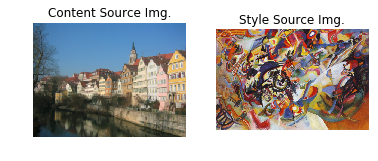Iteration 0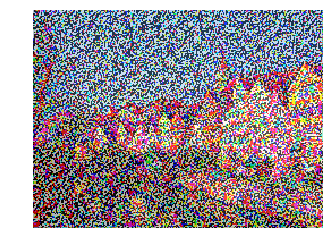Iteration 100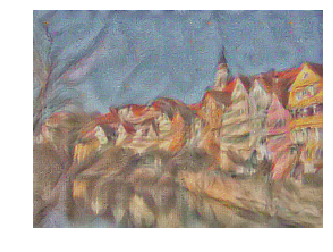Iteration 199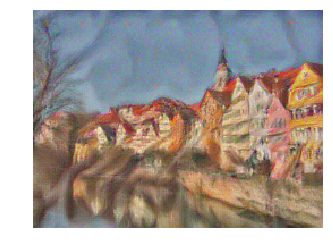In :
# Scream + Tubingen
params2 = {
'content_image':'styles/tubingen.jpg',
'style_image':'styles/the_scream.jpg',
'image_size':192,
'style_size':224,
'content_layer':3,
'content_weight':3e-2,
'style_layers':[1, 4, 6, 7],
'style_weights':[200000, 800, 12, 1],
'tv_weight':2e-2
}

style_transfer(**params2)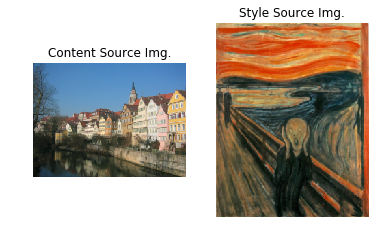Iteration 0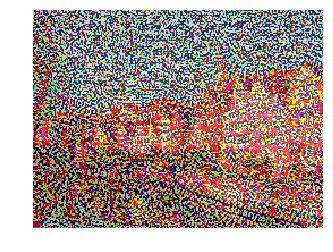Iteration 100